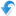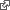## Fuzzy Mortality Model Based on the Algebra of Oriented Fuzzy Numbers

Agnieszka Rossa , University of Lodz
Andrzej Szymanski, University of Lodz

Since the introduction of the Lee-Carter model in 1992 proposed to forecast the trend of age-specific mortality rates, a range of mortality models have been proposed with modeling target defined as the probability of death, the age-specific mortality rate or the force of mortality. The main difficulty in the use of the Lee-Carter model and its stochastic modifications is due to the assumed homogeneity of the random term. However, this property is not confirmed by the analysis of the real-life data. The problem prompted search for solutions that could do without this assumption. One of the possible options is to set research in the framework of the fuzzy number theory. This line of thinking was adopted by Koissi and Shapiro in 2006, where empirical observations and the model's parameters of the Lee-Carter model were treated as fuzzy symmetric triangular numbers. The Koissi-Shapiro model involves some problems with parameter estimation, though, which arise from the necessity to find the minimum of a function with a max-type operator and cannot be solved using standard optimization algorithms. One approach to such a problem can be the Banach algebra of oriented fuzzy numbers OFN, developed by Kosinski, Slezak and Prokopowicz (2003, 2004). In the paper the fuzzy mortality model obtained by applying the OFN algebra to the Koissi Shapiro model will be presented. Prediction accuracy of the proposed model with analogous results obtained with the use of the Lee-Carter model will be also discussed.The research was supported by a grant from the National Science Centre, Poland, under contract UMO-2015/17/B/HS4/00927.See extended abstractPresented in Session P2. Poster Session Ageing, Health and Mortality